# Searchbased Path Planning with Homotopy Class Constraints Subhrajit

• Slides: 20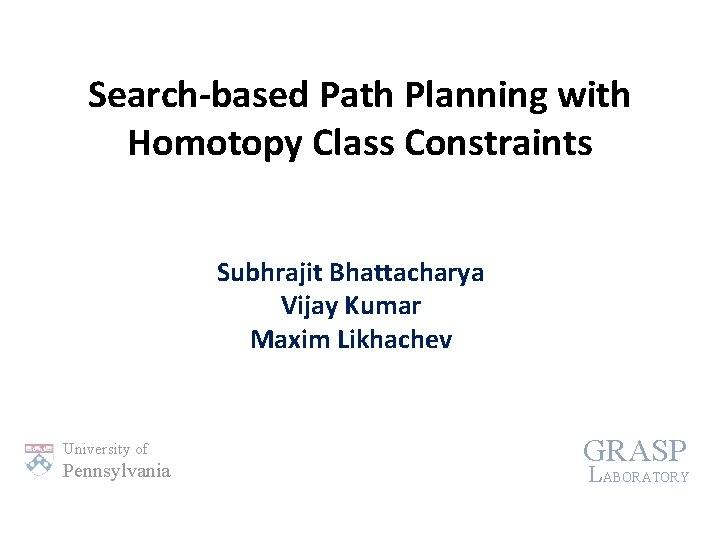Search-based Path Planning with Homotopy Class Constraints Subhrajit Bhattacharya Vijay Kumar Maxim Likhachev University of Pennsylvania GRASP LABORATORY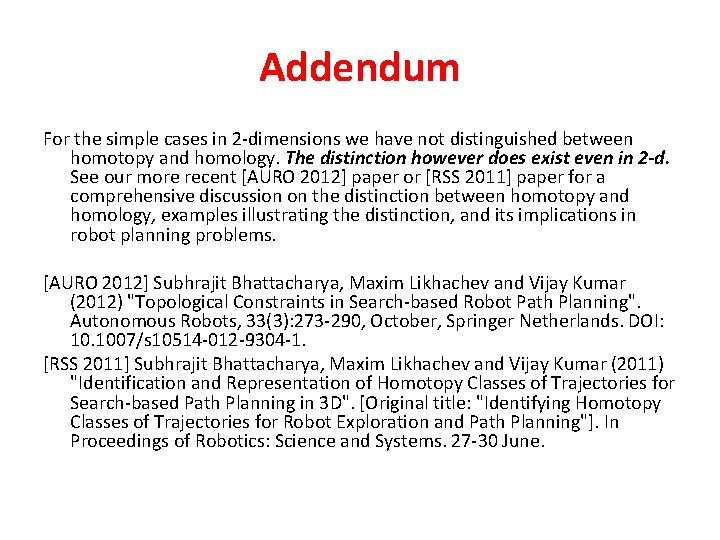Addendum For the simple cases in 2 -dimensions we have not distinguished between homotopy and homology. The distinction however does exist even in 2 -d. See our more recent [AURO 2012] paper or [RSS 2011] paper for a comprehensive discussion on the distinction between homotopy and homology, examples illustrating the distinction, and its implications in robot planning problems. [AURO 2012] Subhrajit Bhattacharya, Maxim Likhachev and Vijay Kumar (2012) "Topological Constraints in Search-based Robot Path Planning". Autonomous Robots, 33(3): 273 -290, October, Springer Netherlands. DOI: 10. 1007/s 10514 -012 -9304 -1. [RSS 2011] Subhrajit Bhattacharya, Maxim Likhachev and Vijay Kumar (2011) "Identification and Representation of Homotopy Classes of Trajectories for Search-based Path Planning in 3 D". [Original title: "Identifying Homotopy Classes of Trajectories for Robot Exploration and Path Planning"]. In Proceedings of Robotics: Science and Systems. 27 -30 June.Homotopy Classes Definition Motivational Example Trajectories in in different same homotopy classses Other applications: ? Deploying multiple agents for: • Searching/exploring the map • Pursuing an agent with uncertain paths ? ? initial goal final ? Path prediction start Avoid or visit certain homotopy classes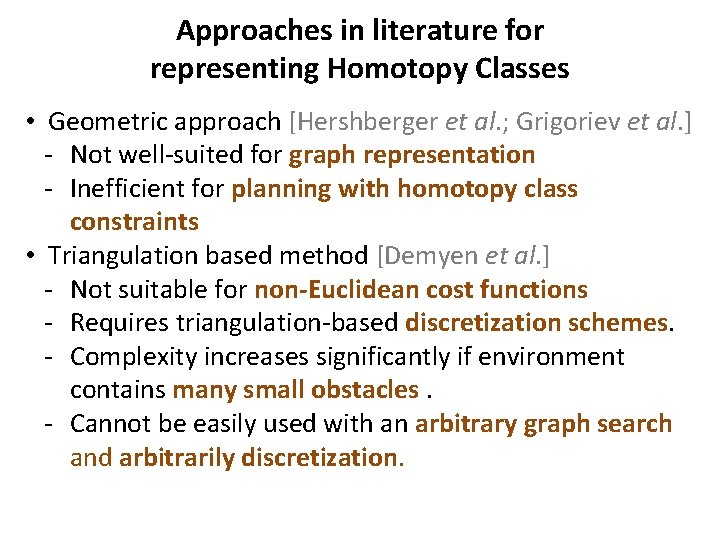Approaches in literature for representing Homotopy Classes • Geometric approach [Hershberger et al. ; Grigoriev et al. ] - Not well-suited for graph representation - Inefficient for planning with homotopy class constraints • Triangulation based method [Demyen et al. ] - Not suitable for non-Euclidean cost functions - Requires triangulation-based discretization schemes. - Complexity increases significantly if environment contains many small obstacles. - Cannot be easily used with an arbitrary graph search and arbitrarily discretization.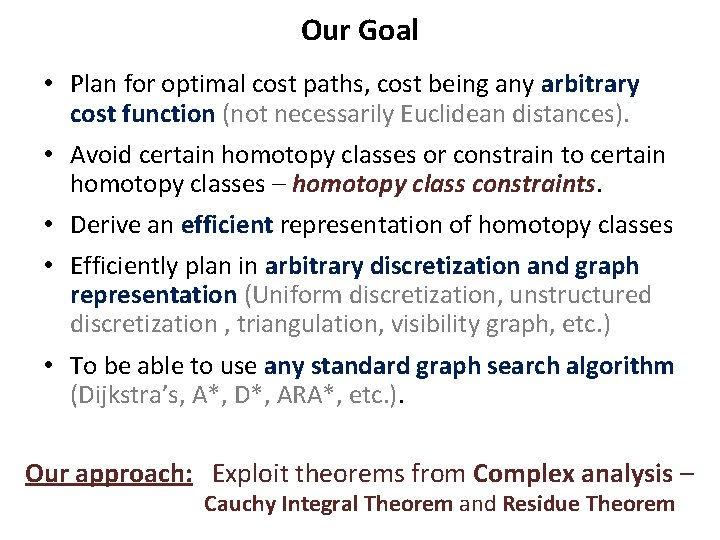Our Goal • Plan for optimal cost paths, cost being any arbitrary cost function (not necessarily Euclidean distances). • Avoid certain homotopy classes or constrain to certain homotopy classes – homotopy class constraints. • Derive an efficient representation of homotopy classes • Efficiently plan in arbitrary discretization and graph representation (Uniform discretization, unstructured discretization , triangulation, visibility graph, etc. ) • To be able to use any standard graph search algorithm (Dijkstra’s, A*, D*, ARA*, etc. ). Our approach: Exploit theorems from Complex analysis – Cauchy Integral Theorem and Residue TheoremBasic Concept (Construction) Im f 0 , for example, can be any arbitrary polynomial in z ζ 1 ζ 2 ζ 3 Re Represent the X-Y plane by a complex plane i. e. A point (x, y) is represented as z = x + iy Place “representative points”, ζi, inside significant obstacles Define an Obstacle Marker function such that it is Complex Analytic everywhere, except for having poles (singularities) at the representative points Complex Analytic Function ≡ Complex Differentiable Functions: F(z) ≡ F (x + iy) ≡ u(x, y) + i v(x, y) x u(x, y) Equivalently, F y = v(x, y) with u, v following certain properties ( 2 u = 2 v = 0) which are guaranteed when x & y are implicitly used within z in construction of F. () ( )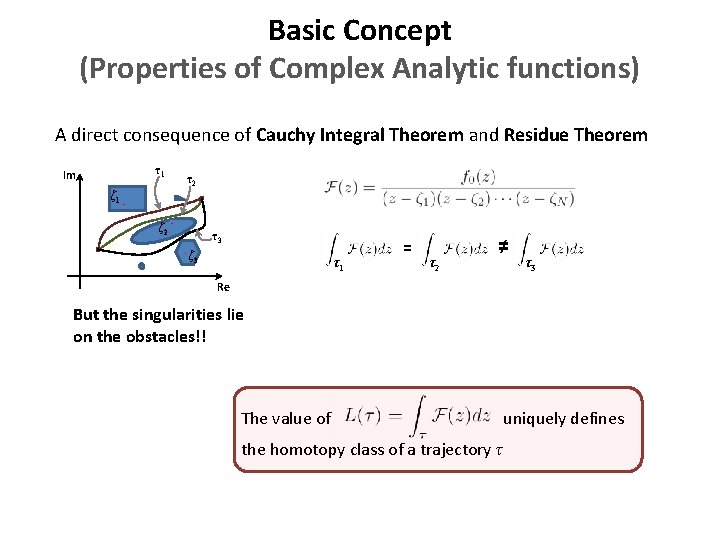Basic Concept (Properties of Complex Analytic functions) A direct consequence of Cauchy Integral Theorem and Residue Theorem τ1 Im τ2 ζ 1 ζ 2 τ3 ζ 3 τ1 = τ2 ≠ τ3 Re But the singularities lie on the obstacles!! The value of the homotopy class of a trajectory τ uniquely defines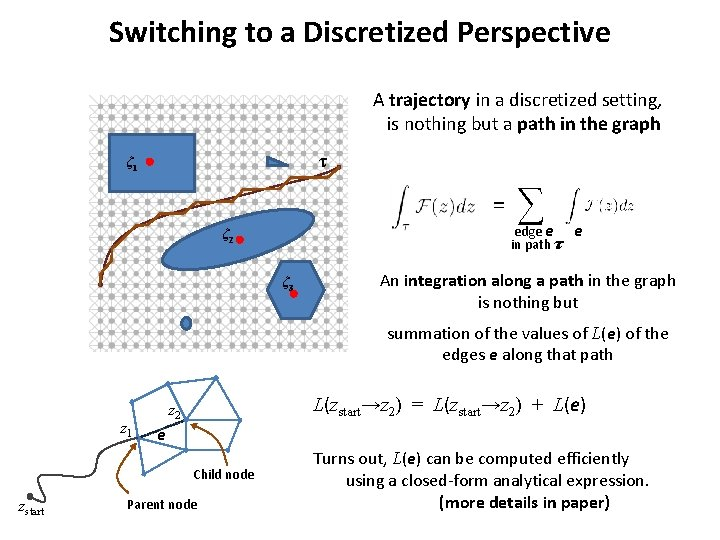Switching to a Discretized Perspective A trajectory in a discretized setting, is nothing but a path in the graph τ ζ 1 = ∑ edge e in path τ ζ 2 ζ 3 e An integration along a path in the graph is nothing but summation of the values of L(e) of the edges e along that path z 1 L(zstart→z 2) = L(zstart→z 2) + L(e) z 2 e Child node zstart Parent node Turns out, L(e) can be computed efficiently using a closed-form analytical expression. (more details in paper)Graph Construction (The L-augmented graph) Given the graph we construct, laid upon the environment, Insight into graph topology: {z, L(zs→z)} in G L z in G z 2 e 1 start zs e 3 ζ 1 e 2 G e 4 More details on Graph construction in paper unique goal state zg z 1 (z 2 , L(e 1)) e 1 start (zs , 0+0 i) GL e 3 ζ 1 e 2 e 4 (z 1 , L(e 2)) (zg , L(e 1)+L(e 3)) ≠ (zg , L(e 2)+L(e 4)) Goal states being distinguished by homotopy class of path taken to reach it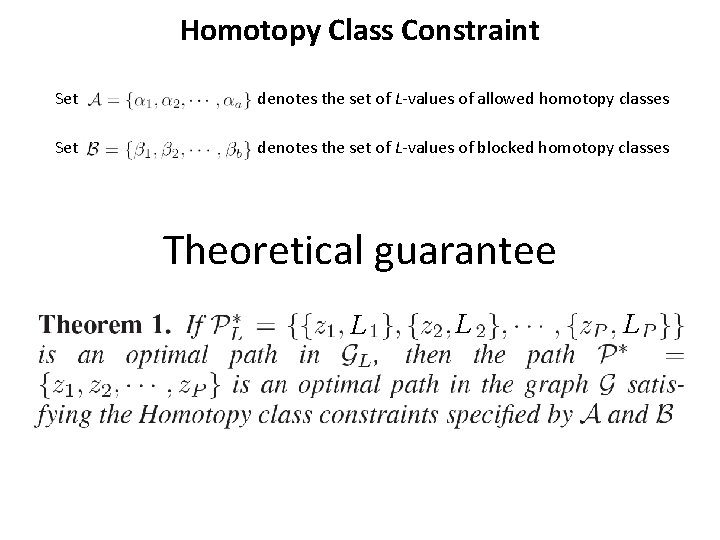Homotopy Class Constraint Set denotes the set of L-values of allowed homotopy classes Set denotes the set of L-values of blocked homotopy classes Theoretical guarantee L L L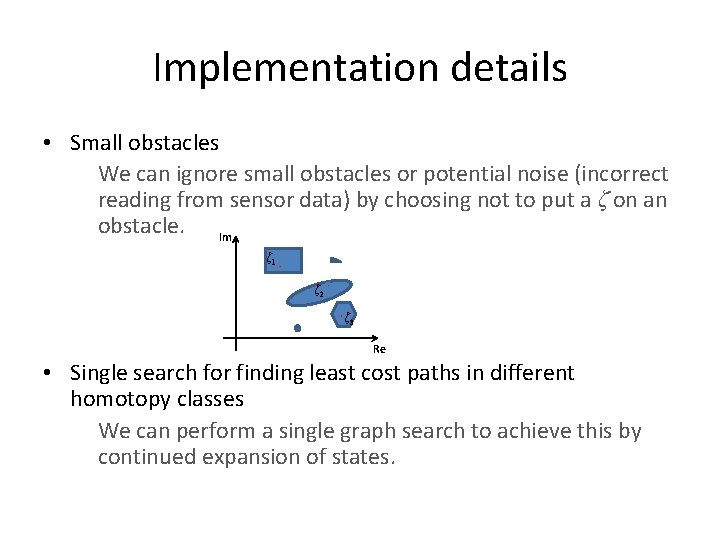Implementation details • Small obstacles We can ignore small obstacles or potential noise (incorrect reading from sensor data) by choosing not to put a ζ on an obstacle. Im ζ 1 ζ 2 ζ 3 Re • Single search for finding least cost paths in different homotopy classes We can perform a single graph search to achieve this by continued expansion of states.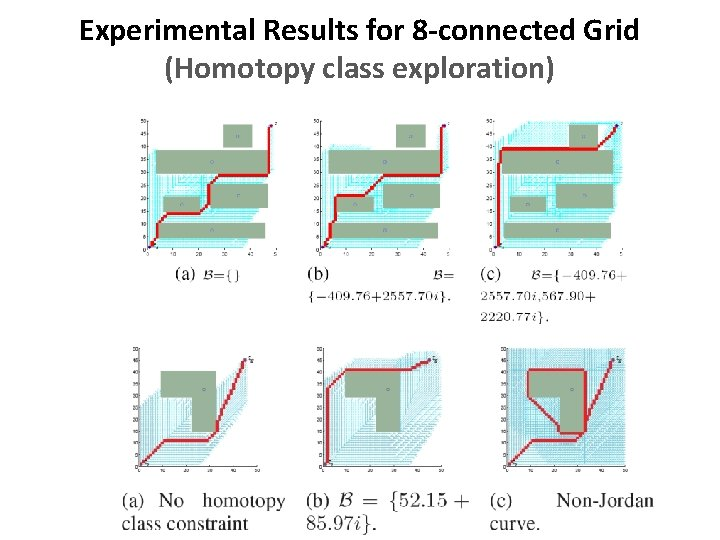Experimental Results for 8 -connected Grid (Homotopy class exploration)Results (“Visibility” constraint translates to homotopy class constraint)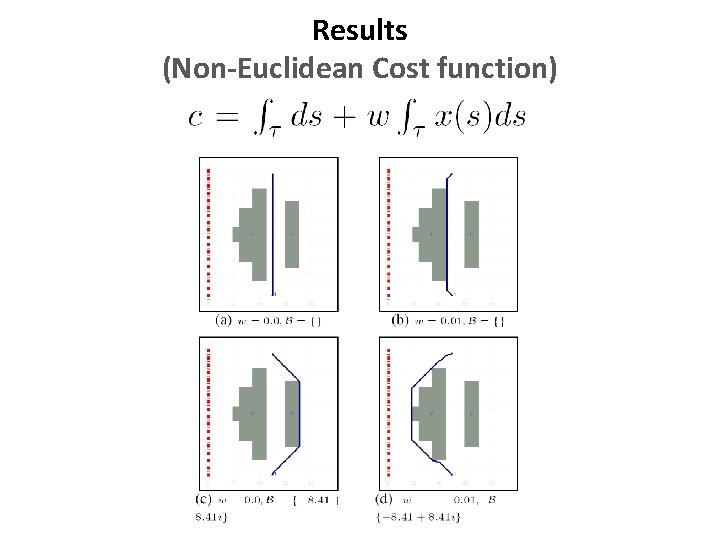Results (Non-Euclidean Cost function)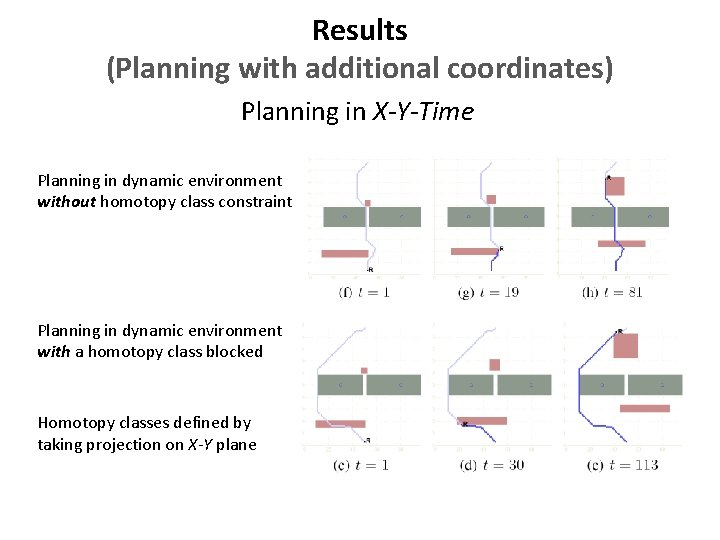Results (Planning with additional coordinates) Planning in X-Y-Time Planning in dynamic environment without homotopy class constraint Planning in dynamic environment with a homotopy class blocked Homotopy classes defined by taking projection on X-Y plane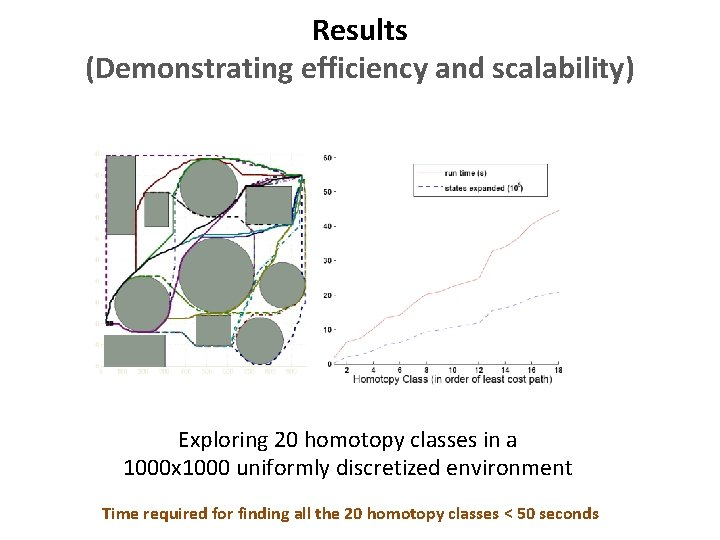Results (Demonstrating efficiency and scalability) Exploring 20 homotopy classes in a 1000 x 1000 uniformly discretized environment Time required for finding all the 20 homotopy classes < 50 seconds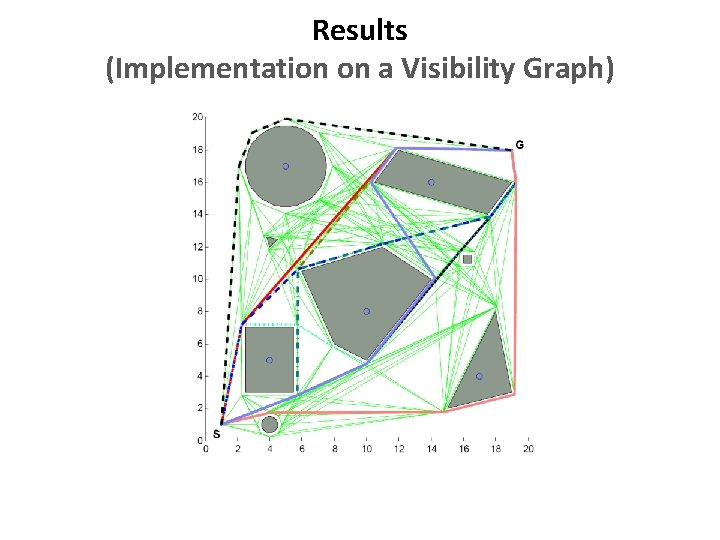Results (Implementation on a Visibility Graph)More interesting results in paperConclusions We have developed a compact and efficient representation of homotopy classes, using which homotopy class constraints can be imposed on existing graph search-based planning methods. Future directions • Extend this method for planning in higher dimensions • Apply the technique for solving more real-life robotics problems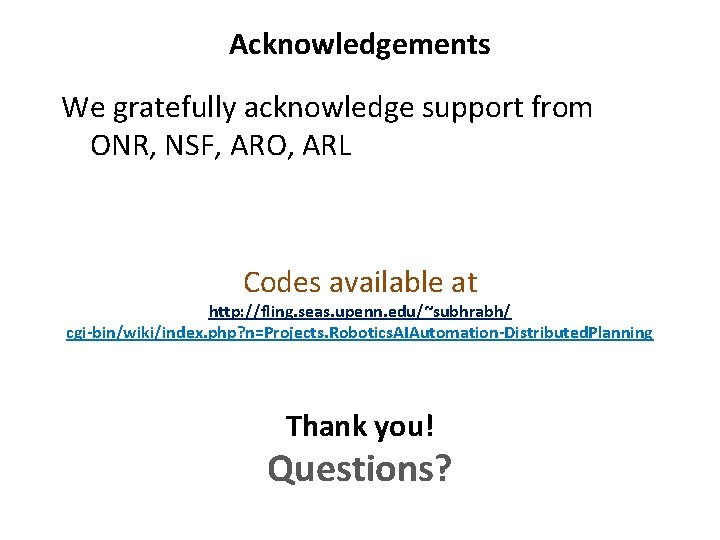Acknowledgements We gratefully acknowledge support from ONR, NSF, ARO, ARL Codes available at http: //fling. seas. upenn. edu/~subhrabh/ cgi-bin/wiki/index. php? n=Projects. Robotics. AIAutomation-Distributed. Planning Thank you! Questions?